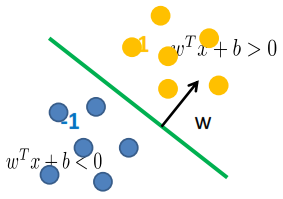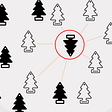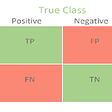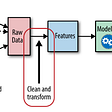# Learning Data Science: Day 11 - Support Vector Machine

We are slowly moving to machine learning topic. In the previous story, we talked about k Nearest Neighbor which categorized as supervised learning. Today, we are going to talk another supervised learning method called Support Vector Machine (SVM).

# Support Vector Machine

Built to handle classification and regression problems, but mostly used in classification problems. In SVM, each data points plotted in n-dimensional space. Where the value of data points coordinates depending on the features. n is specified by the number of features used in the classifier. To classify the data points, SVM will find a hyperplane that differentiates the two classes well.

## Separating Hyperplane

When SVM creating a hyperplane basically there are four things to considers.

• x, are the data points
• y, are the labels of the classes
• w, is the weight vector
• b, is the bias

x are the data points that we have. In image classification x is the set of available images, for other cases that would be different. y, on the other hand, is the class labels. An example in image classification would be when the SVM should define which image have a cat in the picture or a dog in the picture, that’s the labels. To define the orientation of the hyperplane, we will need to use w or also called the weight vector. The main goal of SVM is to estimate the optimal weight vector.

So, we would have something like the image above. Where the blue dotted points is a class, and the yellow one is another class. Some library called the class under the hyperplane function as -1 class, and the class over the hyperplane function as +1 class. Yet, if we only have x, y, and w we would have something like the image below.

Because we only have w, the hyperline is bound to go through the origin of the coordinate system. So, to make it more flexible we can use b or bias to shift the hyperplane around.

And now we will get the function of the hyperplane as the function below.

Now we can define the class of a particular data point based on the hyperplane function. If the function for a data point is less than 0 it will go to -1 class, if the function for a data point is more than 0 it will go to +1 class.Illustration of data points that go to -1 class and +1 class with different color

# Maximum Margin Classification

When choosing the hyperplane it is best to follow the maximum margin classification rule. The margin is the distance between the closest dot point(s) for each class to the hyperplane. The closest data point(s) are the one that we called as the support vectors.

Let’s try it on the picture above. They are an exact same data points, only with different hyperplane function. If we follow the maximum margin rule, we would choose the left one as it is the better one for SVM. If we choose the right one, the margin between the yellow support vector and the hyperplane is too small. So, that if other data points that belongs to yellow came in and were on the left of the support vector of the yellow class then it would create a miss-classification.

# Outliers

Compared to kNN, SVM is able to handle outliers pretty well. By letting the support vector machines to cut some slack.

Basically, slack variables measures the distance between the outliers to the margin where they actually should be placed on the opposite side.

We can actually ignore those outliers compared to kNN that is sensitive to outliers.

# XOR Problem

In some scenario, you wouldn’t be able to create a single linear hyperplane to classify the data points. Such as the picture below.

We can take the SVM to another level by adding an additional dimension. This is what will happen if we add an additional dimension (applying a square function).

Now, when the problem already solved. We can decrease the dimension and we will have a good boundary line that classifies the classes well.

# Wrap Up

So, today we have covered up the basic theory of SVM. However, there might be things that I got it wrong. So, let me know on the response below and we can discuss that. Hopefully, this story may help you and see you on the next story.

--

--

--

## More from Haydar Ali Ismail

Half Data Engineer, Half Software Engineer

Love podcasts or audiobooks? Learn on the go with our new app.

## What interesting insights can we draw from FIFA World Cup Data? Part 2: EDA & Visualization## Data Preprocessing | Practical 2## Untold Story of Results and Discussion Parts of a Manuscript## Sentimental Analysis using Amazon fine food review dataset !!!## 3 Top Python Package to Learn Math for Data Scientist## Delivering Predictive Models to Make Value from Data Science## How I have become Microsoft Certified: Azure Data Scientist Associate## How Machine Learning Works — with Code Example## Haydar Ali Ismail

Half Data Engineer, Half Software Engineer

## K-Nearest Neighbors Classification## Understanding the main classification metrics in machine learning## Difference Between Data Science And Machine Learning With Python?## Getting started with EDA and Feature Engineering